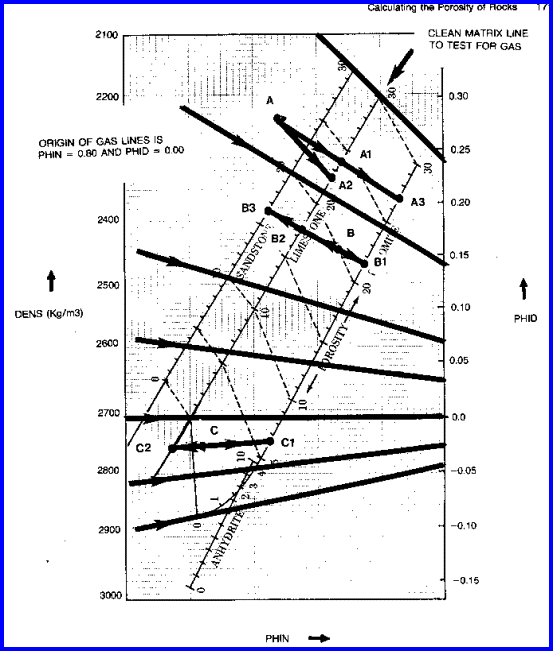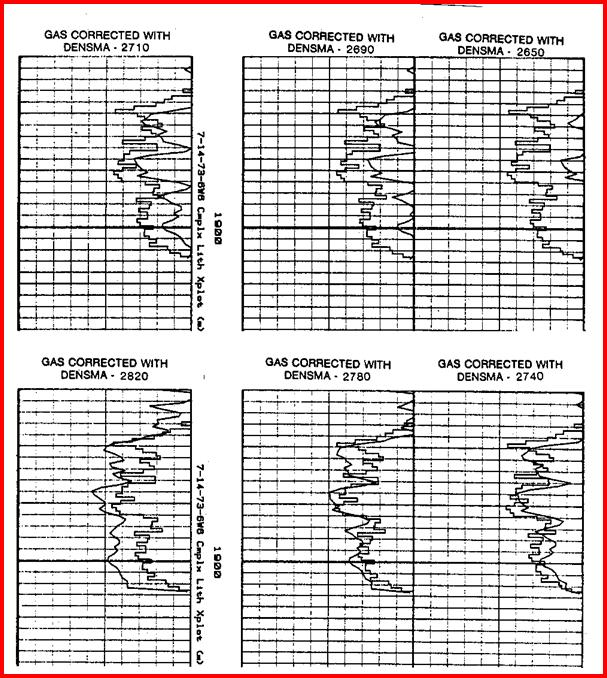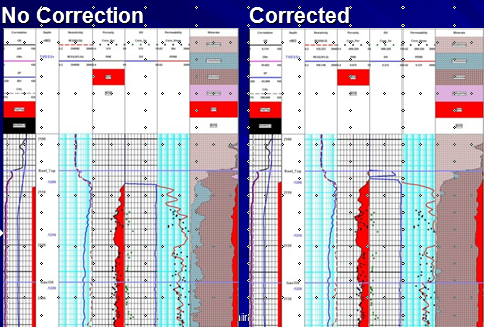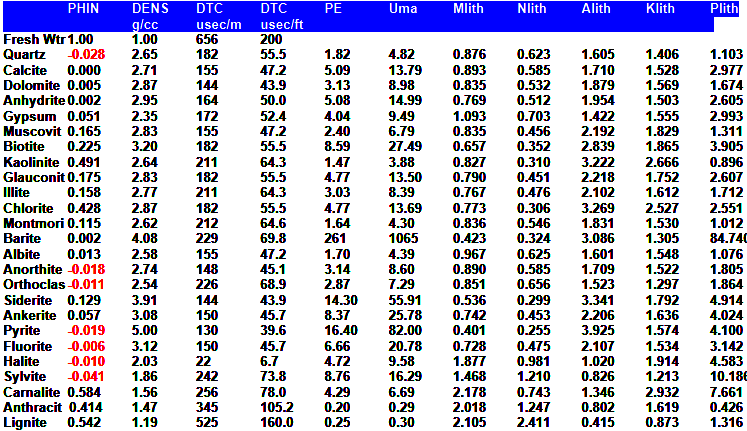Density Neutron  Complex Lithology MODEL
The best method available for modern, accurate, porosity log analysis involves the density neutron crossplot. Several variations on the theme are common, but not all models are recommended. A crossplot method, called the shaly sand model was once widely used. It was found to be a poor model for any sandstone that contained other minerals in addition to quartz.

The shale corrected density neutron complex lithology crossplot model works in quartz/feldspar as well as in other mineral mixtures without any prior knowledge of mineralogy, so it is the preferred model today. The only limiting factor is that both logs need to be available and the density log do not suffer from rough hole effects over the zone of interest.
Although the name of the method is complicated, the mathematics are not.Density Neutron Crossplot POROSITY Complex Lithology model
STEP 1: Shale correct the density and neutron log data for each layer:

1: PHIdc = PHID – (Vsh * PHIDSH)

2: PHInc = PHIN – (Vsh * PHINSH)

PHIDSH and PHINSH are constants for each zone, and are picked only once.

STEP 2: Check for gas crossover after shale corrections and calculate porosity for each layer from the correct equation:

3: IF PHInc >= PHIdc, there is no gas crossover

4: THEN PHIxdn = (PHInc + PHIdc) / 2

The density neutron crossplot porosity, PHIxdn, after all corrections are applied, is called the effective porosity, PHIe.Density Neutron Complex Lithology Crossplot - Oil and Water cases,
or Gas zones with crossover.

Chartbook solutions are provided above. Shale corrected data must be entered.COMMENTS
Use in preference to most methods if data is available, even in shaly sands to correct for heavy mineral content.

·      Do not use when density is affected by bad hole conditions.

·      No correction for log units (eg Sandstone or Limestone units) is needed for most cases, except gas in dolomite and low porosity dolomite. Use Limestone units log ONLY for these two special cases (See below).

·      Shale corrections could create apparent gas crossover and this may be real or an artifact of excessive correction. Check against known data from the well if shale correction creates crossover.

·      To calibrate to core porosity, adjust PHIDSH, PHINSH, or Vsh to obtain a better match by trial and error. Appropriate crossplots may assist, or regression of PHIxdn vs core porosity may be used.SPECIAL CASES - GAS CORRECTIONS:

CASE 1: IF gas is known to be present AND gas crossover occurs after shale corrections, apply the following gas correction:

6: IF PHInc < PHIdc, there is gas crossover

7: THEN PHIxdn = ((PHInc ^ 2 + PHIdc ^ 2) / 2) ^ 0.5

CASE 2: IF gas is known to be present but NO crossover occurs after shale corrections, this usually means gas in dolomite or in a sandstone with lots of heavy minerals. First, assume or calculate the matrix density

(DENSMAgc) based on the PE curve (PE is the only curve unaffected by gas):
8: V1 = (PE - Vsh * PESH - PE2) / (PE1 - PE2)
9: V2 = 1 - V1
10: DENSMAgc = V1 * DENS1 + V2 * DENS2

Where:
DENSMAgc =  matrix density for gas correction (kg/m3 or gm/cc)
DENS1 = matrix density of mineral 1 (kg/m3 or gm/cc)
DENS2 = matrix density of mineral 2 (kg/m3 or gm/cc)
PE = measured PE log value of rock mixture
PE1 = PE of first mineral (fractional)
PE2 = PE of second mineral (fractional)
V1 = volume of first mineral (fractional)
V2 = volume of second mineral (fractional)
Vsh = volume of shale (fractional)

DENSMAgc can be computed as a continuous curve or used as a zone parameter to replace DENSMA in equation 8.

Apply gas correction:

11: PHIx = – PHIdc / (PHInc / 0.8 – 1) / (1 + PHIdc / (0.8 – PHInc))

12: PHIxdn = PHIx + KD3 * (0.30 – PHIx) * (DENSMAgc / KD1 – KD2)

Where:  KD1 = 1.00 for English units

KD1 = 1000 for Metric units

KD2 = 2.65 for Sandstone scale log

KD2 = 2.71 for Limestone scale log

KD3 = 1.80 for Sandstone scale log

KD3 = 2.00 for Limestone scale logDensity Neutron Complex Lithology Crossplot - Gas zones with NO crossover. Enter shale corrected data and then slide data point to the right until it reaches the line representing the matrix density of the reservoir - travel parallel to the nearest heavy black line. Do not use Dolomite scale log for this special case.

An illustration of how the points move under different conditions is given below.Graph for Gas Correction on Density Neutron Crossplot Porosity, showing how the raw data points
move under various assumptions. (Porosity scales are in Limestone Units)

Point A goes to A1, if CNL and DENSMA = 2710 (crossover)
goes to A2, if SNP regardless of DENSMA
goes to A3, if CNL and DENSMA = 2870.

Point B goes to B1, if CNL and DENSMA = 2870 and gas correction is IMPOSED
goes to B2, if CNL and DENSMA = 27l0 and gas correction is IMPOSED
goes to B3, if CNL and DENSMA = 2650 and gas correction is IMPOSED
stops at B if gas correction is not imposed or if SNP

Point C goes to C1, if CNL and DENSMA = 2870 and gas correction is IMPOSED
goes to C2, if CNL and DENSMA = 2710 and gas correction is IMPOSED
stops at C if gas correction not imposed or if SNP

The author is indebted to Jim Hamilton of Dome Petroleum for first suggesting this approach to one pass gas corrections. The approach has proved extremely successful and has matched core on hundreds of projects in many reservoir conditions.

Before the introduction of the photo-electric effect (PE) log curve, it was easy for a log analyst to miss a gas filled dolomite reservoir. The standard density neutron crossplot would show a low porosity limestone, when in fact the zone is a medium porosity dolomite. Since the density neutron looks like limestone (curve separation is small) and the PE looks like dolomite (PE near 3.0), this discrepancy is a red flag that a special case exists. Many computer programs will not trigger gas corrections unless density neutron crossover is present, and most programs do not contain explicit algorithms to handle this special case.

The illustration below  shows the effect of using this gas correction. Notice that computed porosity does not match core porosity unless the correct DENSMA is chosen. DENSMA should reflect the matrix density of the expected lithology. The correction is usually needed in dolomite or dolomitic sands or high porosity shaly sands with low to moderate invasion.Effect of DENSMA on density neutron crossplot porosity with gas in heavy minerals. Core porosity (square black lines) and log analysis porosity (smooth black curves) show a good match when DENSMA was set at
2710 - 2740 kg/m3. Log analysis shows near zero porosity if DENSMA set at 2650 for this heavy sandstone.Gas in dolomite example with no correction applied (left) and after correction (right).SPECIAL CASES - LOW POROSITY and SECONDARY POROSITY:
CASE 3: IF rock is dolomite AND porosity is less than 0.05, use the following instead of Equation 4:

13: E = (4 - (3.3 + 10 ^ (-5 * PHInc - 0.16))

14: PHIxdn = (E * PHIdc + 0.754 * PHInc) / (E + 0.754)

Log data must be in Limestone Units for this Case. This option can be used instead of equation 4 as long as there is no gas crossover after shale corrections. It is slightly more accurate, but requires a computer or preprogrammed calculator. Some more modern CNL logs use alternate porosity transforms and are more linear at low porosities, so this fix may not be needed (rg. TNPH curve).

CASE 4: IF Archie or dual water model is to be used for water saturation, the following is needed:

15: BVWSH = (PHIDSH + PHINSH) / 2     (a constant for the zone)

16: PHIt = (PHID + PHIN) / 2                      (one value for each layer)

CASE 5: IF zone is vuggy carbonate, calculate secondary porosity:

17: PHIsec = PHIxdn - PHIscDensity LOG PARAMETERSPage Views ---- Since 01 Jan 2015
Copyright 2023 by Accessible Petrophysics Ltd.
CPH Logo, "CPH", "CPH Gold Member", "CPH Platinum Member", "Crain's Rules", "Meta/Log", "Computer-Ready-Math", "Petro/Fusion Scripts" are Trademarks of the Author